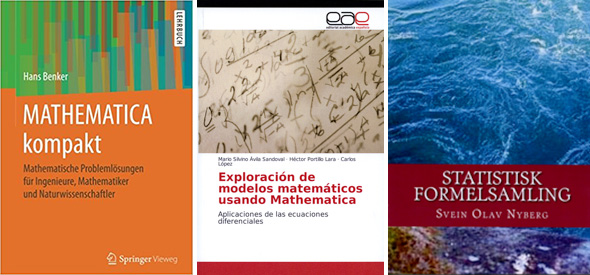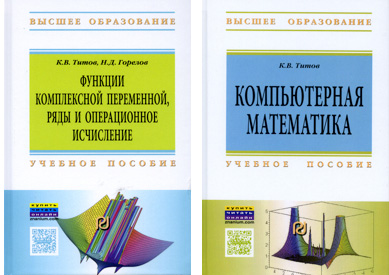Browse by Topic
Related Topics

# Books from around the (Wolfram) World!

We’re always excited to see what new things people have created using Wolfram technologies. As the broad geographical distribution of Wolfram Community contributors illustrates, people all over the world are doing great things with the Wolfram Language. In this vein, today we want to highlight some recent books written in languages other than English that utilize Wolfram technologies. From engineering to statistics, these books provide valuable information for those looking to dig a little deeper into scientific applications of the Wolfram Language.Hans Benker provides a brief and accessible introduction to Mathematica and shows its applications in problems of engineering mathematics, discussing the construction, operation and possibilities of the Wolfram Language in detail. He explores Mathematica usage for matrices and differential and integral calculus. The last part of the book is devoted to the advanced topics of engineering mathematics, including differential equations, transformations, optimization, probability and statistics. The calculations are all presented in detail and are illustrated by numerous examples.

This book explores mathematical models that are traditionally studied in courses on differential equations, but from a unique perspective. The authors analyze models by modifying their initial parameters, transforming them into problems that would be practically impossible to solve in an analytical way. Mathematica provides an essential computational platform for solving these problems, particularly when they are graphical in nature.

Svein Olav Nyberg provides an undergraduate-level statistical formulary with support for Mathematica. This volume includes basic formulas for Bayesian techniques, as well as for general basic statistics. It is an essential primer for Norwegian-language students working in statistical analysis.Computational thinking is an increasingly necessary technique for problem solving in a range of disciplines, and Mathematica and the Wolfram Language equip students with a powerful computational tool. Approaching calculus from this perspective, K. V. Titov and N. D. Gorelov’s textbook provides a helpful introduction to using the Wolfram Language in the mathematics classroom.

Another textbook from K. V. Titov, Kompyuternaya matematika: uchebnoe posobie emphasizes the use of computer technologies for mathematical analyses and offers practical solutions for numerous problems in various fields of science and technology, as well as their engineering applications. Titov discusses methodological approaches to problem solving in order to promote the development and application of online resources in education and to help integrate computer mathematics in educational technology.

These titles are just a sampling of the many books that explore applications of the Wolfram Language. You can find more Wolfram technologies books, both in English and other languages, by visiting the Wolfram Books site.